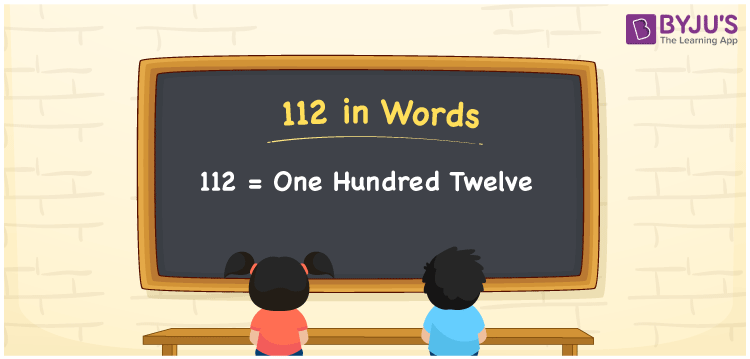# 112 in Words

112 in English words can be written as One Hundred Twelve. The number name of 112 is written using the ones, tens, hundreds places of a number. Thus, a place value chart is helpful in writing any numbers in words. For example, If you bought chocolates for Rs.112, you can write “I bought chocolates worth Rupees One Hundred Twelve”. 112 is a cardinal number as it shows a specific amount. Let us learn the spelling of 112 in this article.

 112 in Words One Hundred Twelve One Hundred Twelve in numerical form 112

## 112 in English Words

Generally, numbers in words are represented using the English alphabet. Thus, students must know English to express the numbers in word form. Hence, 112 in words is written as One Hundred Twelve.## How to Write 112 in Words?

To express 112 in words, first identify the place value of each digit in a number. Let us make a chart with three columns as 112 is a three-digit number.

 Hundreds Tens Ones 1 1 2

Thus, we can write the expanded form as:

1 x Hundred + 1 x Ten + 2 x One

= 1 x 100 + 1 x 10 + 2 x 1

= 100 + 10 + 2

= 112

= One Hundred Twelve

Hence, 112 in words is written as One Hundred Twelve.

Interesting way of writing 112 in words

1 = One

11 = Eleven

112 = One Hundred Twelve

Thus, the word form of the number 112 is One Hundred Twelve.

112 is a natural number whose successor is 113 and the predecessor is 111

• 112 in words – One Hundred Twelve
• Is 112 an odd number? – No
• Is 112 an even number? – Yes
• Is 112 a perfect square number? – No
• Is 112 a perfect cube number? – No
• Is 112 a prime number? – No
• Is 112 a composite number? – Yes

## Frequently Asked Questions on 112 in Words

Q1

### How to write 112 in words?

112 in words is written as One Hundred Twelve.
Q2

### What is the value of 50 plus 62?

50 + 62 = 112 Hence, the value of 50 plus 62 is 112.
Q3

### 112 is a prime number. True or False.

False, 112 is not a prime number.
Test your Knowledge on 112 in Words